Geometría proyectiva: Application of second-order overlapping beamsProjective concepts that we have developed in studying the overlapping beams of second order, whose base is a conical, They allow to solve problems of determination of points of contact in the tangent of a Conic defined by five tangent or five restrictions through the combination of tangent and with their respective tangent points.

To solve this type of problems we will remember that given two beams of second order, When sectioning them from two homologous elements are obtained perspectives series that projected from the projective center beams (Brianchon point). In the following figure, the homologous points a-a.’ They determine the double point of the perspectives series, While the AB'-to 'B-AC' to ' C projected the straight lines 1 and 2 that contain your prospective Center respectively (“V..” It is the projective Center of bundles of second order mentioned above)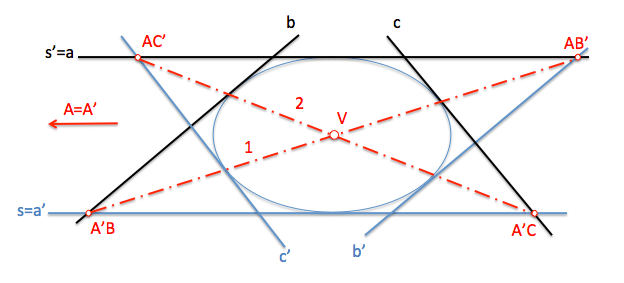General model for Brianchon point

Homologous rays that serve as bases for these perspectives series can be any of the three pairs that define the is between the beams of the second order. We can see that if we cut from all of them we get three straight (1,2 and 3) containing it to the Brianchon point, from which the double lines be drawn (tangent if any) beams (It will be imaginary if this point is the conical interior).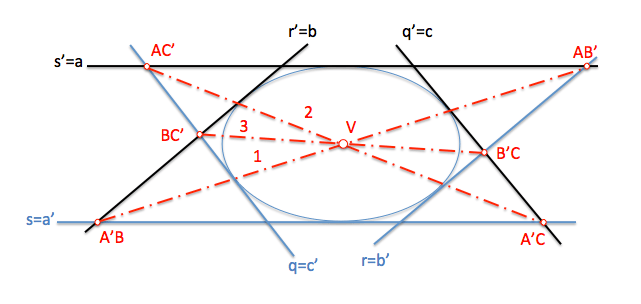Center of Brianchon point of tangency

The exposed projective model allows to relate the tangent of the cone with their points of tangency, thinking that a point of tangency is the intersection of two tangent infinitely close.

For example, If we move the tangent line “c” from the figure above to match the straight line “b'” keeping the geometric constraints of this figure, We will have to b-c’ It has become a point of tangency which will belong to the straight “3” passing through the Centre of the projective “V..”.

Brianchon point with two points of tangency

Matching a second pair of tangents as b-c’ (It could also be a-c’ or ’-c) We will obtain a variant of the previous model, but in this case with two points of tangency.

Brianchon point with three points of contact

If we do agree to two tangent three, for example a-c ', b-a’ and c-b ’, We will have three points of tangents in this variant of the general model. You can use other combinations of the tangents, but will have to wear each pair one of each of the beams and in any case two counterparts (as a-a. ’, b-b’ or c-c ’).

Statement of issues

These figures allow us to pose problems of determination of points of contact in the tangents that determine the Conic as shown in an example, the reader leaving the resolution of the remaining.

The problems that can arise, understanding the Conic as envelope of the tangent, its:

1. Given a Conic tangent five, determine the point of tangency on one of them.
2. Given a tangent with its point of contact and three additional tangent of a conical, determine the point of contact in another of the tangents.
3. Given two tangent with their respective points of contact and an additional tangent, determine the contact point is tangent.

Application to problem solving

We will resolve the first of the problems raised as an example:

Given the straight lines p, q, r, s and t tangent to a conical, determine the point “T” contact the straight “t“.1.-Determination of the figure of analysis of application

We will use as a figure of analysis to solve the problem that we have tagged as “Brianchon point with a point of tangency”, as in this variant of the “General model” We have a point of contact in one of the tangents.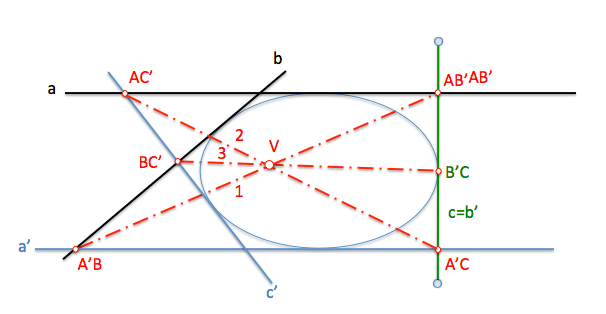2.- Allocation of the corresponding labels

We shall first proceed to identify the straight lines of the wording of the problem with the tangent to the Conic Figure analysis, taking into account that, in this case, We assign each second-order beam straight to the straight “t” in which we want to find the point of contact.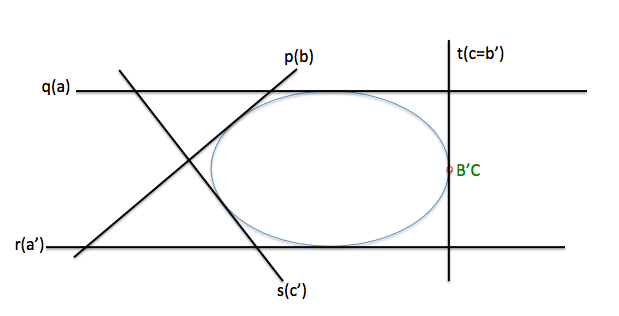3.- Determination of the is

Once determined the elements of the beams, We obtain the projective Center them (Brianchon point).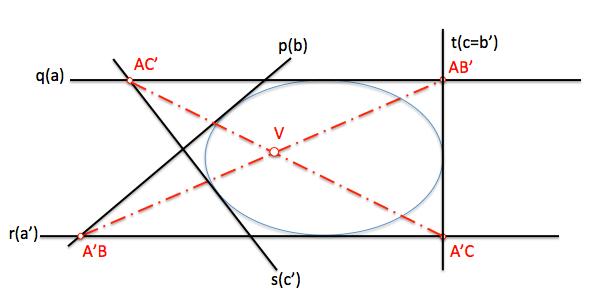4.- Resolving the problem

Finally determine the tangency point knowing that this, point B'C, It will be screened from the projective Center with its homologous point BC’Similarly, we solve the two remaining cases.

Can you solve them?